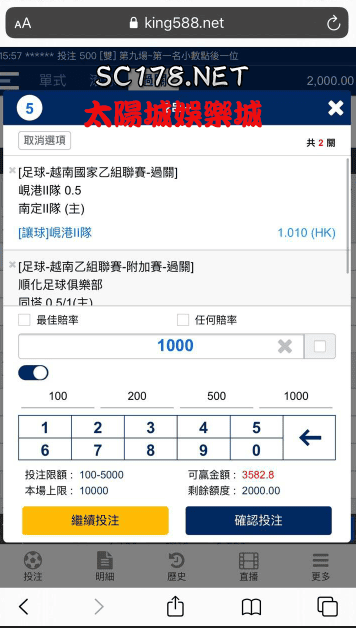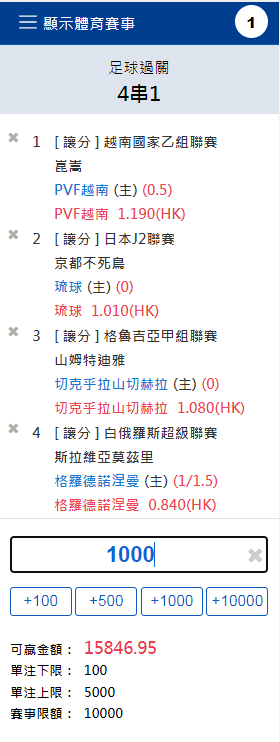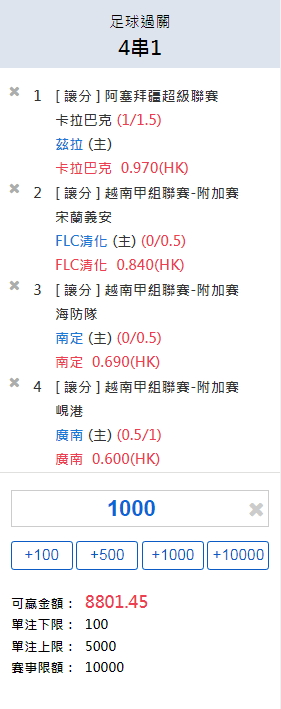## 運彩過關計算|三分鐘教你看懂運彩過關怎麼算1000×（1+1.190)×(1+1.010)×(1+1.080)×(1+0.840)=168461000×(1+0.970÷2)×(1+0.840)×(1+0.690)×(1+0.600)=49001000÷2×(1+0.970)×(1+0.840)×(1+0.690)×(1+0.600)=4900

(0.8+1)×(0.9+1)×(0.96+1)×1000-1000=4660元。

（(0.8+1)×0.5）×(0.9+1)×(0.96+1)×1000-1000=2351。

（(0.9+1)×(0.96+1)×1000-1000）×0.5=1326。